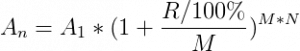# Compound Interest Calculator

Enter the initial amount, annual interest rate, period, then click the Calculate button to get the result.

## Compound interest calculation formula:A1 – initial amount
An – amount after n years (or days/weeks/months)
R – nominal annual interest rate
M – number of compounding periods in one year
N – number of years (or days/weeks/months)